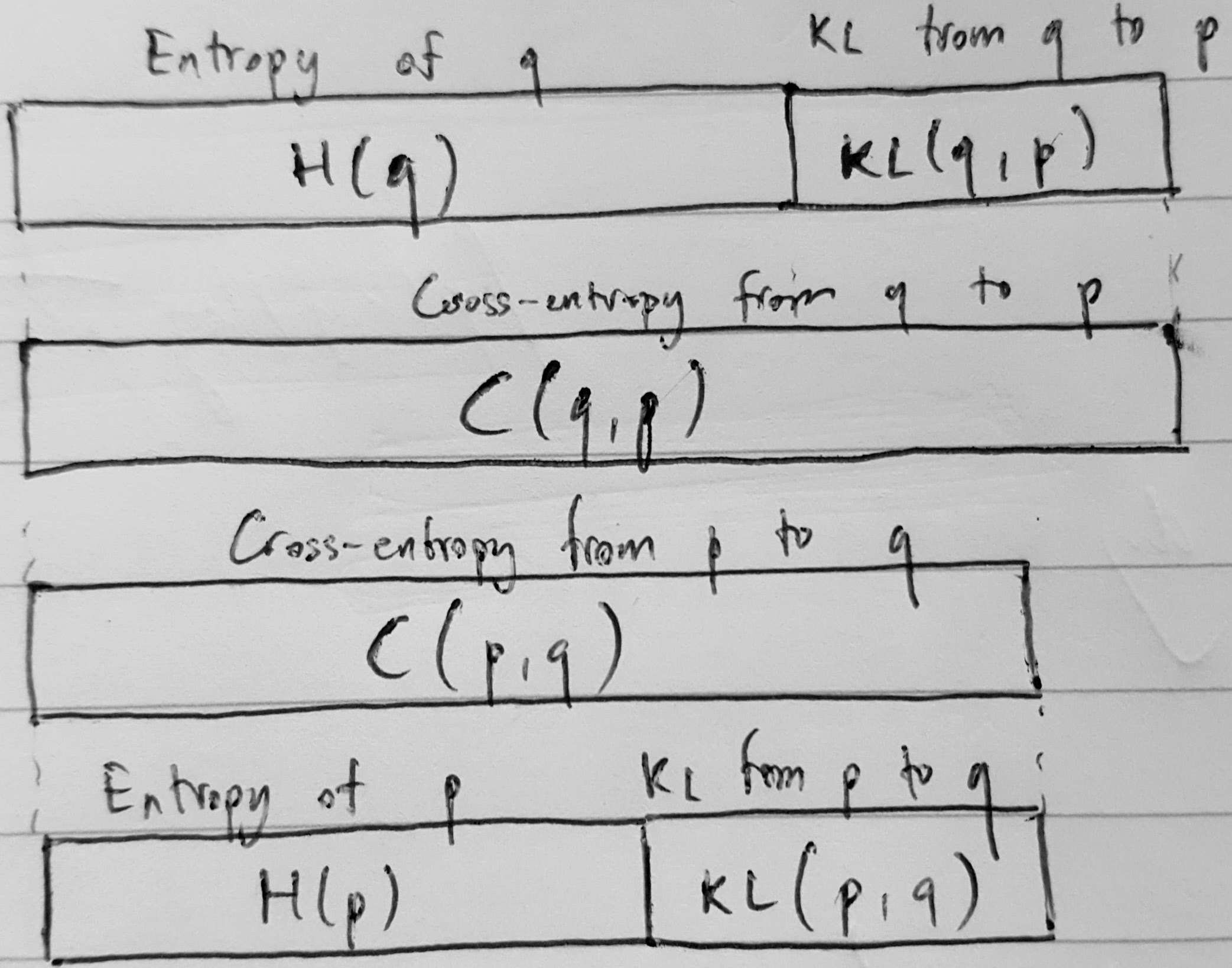# information theory basics

04 April 2017

goal: build some intuition for basics of information theory.

## one random variable

entropy of a random variable $$X: \Omega \to \mathcal X$$ with a density $$p(x)$$ with respect to the base measure $$\mathrm dx$$ is \begin{align} H(p) = \int p(x) \log \frac{1}{p(x)} \,\mathrm dx. \end{align} it measures uncertainty in $$X$$. the higher the entropy, the more “uncertainty” there is. it represents, among other things, the minimum average code length for communicating $$X$$ using variable-length code when $$\mathcal X$$ is discrete. in other words, if we had a mismatched model for $$X$$ which had the density $$q(x)$$, the average code length for communicating $$X$$ would be higher than $$H(p)$$. this quantity is referred to as the cross-entropy: \begin{align} C(p, q) = \int p(x) \log \frac{1}{q(x)} \,\mathrm dx, \end{align} where \begin{align} C(p, q) \geq H(p). \end{align} the difference between $$C(p, q)$$ and $$H(p)$$ is called the KL-divergence from $$p$$ to $$q$$: \begin{align} \mathrm{KL}(p, q) &= C(p, q) - H(p) \\
&= \int p(x) \log\frac{p(x)}{q(x)}. \end{align} the KL-divergence is $$\geq 0$$ with the equality holding only if $$p = q$$.

conversely, \begin{align} H(q) &= \int q(x) \log \frac{1}{q(x)} \,\mathrm dx \\
C(q, p) &= \int q(x) \log \frac{1}{p(x)} \,\mathrm dx \\
\mathrm{KL}(q, p) &= \int q(x) \log \frac{q(x)}{p(x)} \,\mathrm dx. \end{align} note that in general, $$C(q, p) \neq C(p, q)$$ and $$\mathrm{KL}(q, p) \neq \mathrm{KL}(p, q)$$.## two random variables

entropy of the joint random variable $$(X, Y)$$ with the density $$p(x, y)$$ is, adopting a different notation from previous section, \begin{align} H(X, Y) = \int p(x, y) \log \frac{1}{p(x, y)} \,\mathrm dx\,\mathrm dy. \end{align}

the conditional entropy of $$X$$ given $$Y = y$$ is \begin{align} \int p(x \given y) \log \frac{1}{p(x \given y)} \,\mathrm dx. \end{align} intuitively, and hand-wavingly, this tells us what’s the minimum average code length for communicating $$X$$ (given the correlation between $$X$$ and $$Y$$ encoded in the joint distribution over them), given that we know that $$Y = y$$.

this quantity is usually averaged over the marginal distribution of $$Y$$, with the density $$p(y)$$. we obtain the conditional entropy of $$X$$ given $$Y$$: \begin{align} H(X \given Y) &= \int p(y) \int p(x \given y) \log \frac{1}{p(x \given y)} \,\mathrm dx \,\mathrm dy \\
&= \int p(x, y) \log \frac{1}{p(x \given y)} \,\mathrm dx \,\mathrm dy \end{align} the reason for averaging this over $$Y$$ is a bit arbitrary the way it is presented. i will need to investigate further, but i suspect this entropy is the entropy of the conditional expectation $$\E[X \given Y]$$ which is also a random variable. for now, let’s just build some sort of intuition. idk how to understand this intuitively.

entropy as a measure of information/uncertainty has been designed to follow the rule of additivity, i.e. joint entropy of two independent random variables should be the sum of the individual entropies of each of them. in this case, we can verify this on the random variables $$X \given Y$$ (or probably more accurately $$\E[X \given Y]$$) and $$Y$$: \begin{align} H(X, Y) - H(X \given Y) &= \int p(x, y) \log \frac{1}{p(x, y)} \,\mathrm dx\,\mathrm dy - \int p(x, y) \log \frac{1}{p(x \given y)} \,\mathrm dx \,\mathrm dy \\
&= \int p(x, y) \left[ \log \frac{1}{p(x, y)} - \log \frac{1}{p(x \given y)} \right] \,\mathrm dx\,\mathrm dy \\
&= \int p(y) p(x \given y) \log \frac{1}{p(y)} \,\mathrm dx \,\mathrm dy \\
&= \int p(y) \int p(x \given y) \,\mathrm dx \log \frac{1}{p(y)} \,\mathrm dy \\
&= \int p(y) \log \frac{1}{p(y)} \,\mathrm dy \\
&= H(Y). \end{align}

similarly, we can derive $$H(X, Y) = H(Y \given X) + H(X)$$ for \begin{align} H(Y \given X) &= \int p(x) \int p(y \given x) \log \frac{1}{p(y \given x)} \,\mathrm dy \,\mathrm dx \\
H(X) &= \int p(x) \log \frac{1}{p(x)} \,\mathrm dx. \end{align}

i don’t know how to prove it, but $$H(X \given Y) \leq H(X)$$ and $$H(Y \given X) \leq H(Y)$$. intuitively, entropy of a random variable given some extra information is not higher than of the same random variable without any extra information.[back]